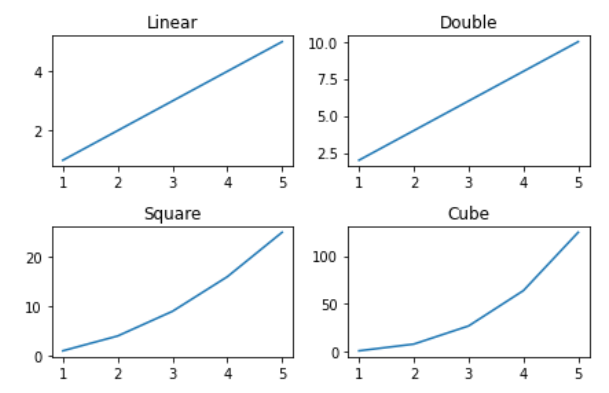# How to Add Title to Subplots in Matplotlib?

In this article, we will see how to add a title to subplots in Matplotlib? Let’s discuss some concepts :

• Matplotlib : Matplotlib is an amazing visualization library in Python for 2D plots of arrays. Matplotlib is a multi-platform data visualization library built on NumPy arrays and designed to work with the broader SciPy stack. It was introduced by John Hunter in the year 2002.
• Subplots : The subplots() function in pyplot module of matplotlib library is used to create a figure and a set of subplots. Subplots are required when we want to show two or more plots in same figure.
• Title of a plot : The title() method in matplotlib module is used to specify title of the visualization depicted and displays the title using various attributes.

### Steps Needed

• Import Libraries
• Make subplot
• Plot subplot
• Set title to subplots.

Example 1: (Using set_title() method)

We use matplotlib.axes._axes.Axes.set_title(label) method to set title (string label) for the current subplot Axes.

## Python3

 `# importing packages` `import` `numpy as np` `import` `matplotlib.pyplot as plt`   `# create data` `x``=``np.array([``1``, ``2``, ``3``, ``4``, ``5``])`   `# making subplots` `fig, ax ``=` `plt.subplots(``2``, ``2``)`   `# set data with subplots and plot` `ax[``0``, ``0``].plot(x, x)` `ax[``0``, ``1``].plot(x, x``*``2``)` `ax[``1``, ``0``].plot(x, x``*``x)` `ax[``1``, ``1``].plot(x, x``*``x``*``x)`   `# set the title to subplots` `ax[``0``, ``0``].set_title(``"Linear"``)` `ax[``0``, ``1``].set_title(``"Double"``)` `ax[``1``, ``0``].set_title(``"Square"``)` `ax[``1``, ``1``].set_title(``"Cube"``)`   `# set spacing` `fig.tight_layout()` `plt.show()`

Output:Example 2: (Using title.set_text() method)

We can also add title to subplots in Matplotlib using title.set_text() method, in similar way to set_title() method.

## Python3

 `# importing packages` `import` `numpy as np` `import` `matplotlib.pyplot as plt`   `# create data` `x``=``np.array([``1``, ``2``, ``3``, ``4``, ``5``])`   `# making subplots` `fig, ax ``=` `plt.subplots(``2``, ``2``)`   `# set data with subplots and plot` `ax[``0``, ``0``].plot(x, x)` `ax[``0``, ``1``].plot(x, x``*``2``)` `ax[``1``, ``0``].plot(x, x``*``x)` `ax[``1``, ``1``].plot(x, x``*``x``*``x)`   `# set the title to subplots` `ax[``0``, ``0``].title.set_text(``"Linear"``)` `ax[``0``, ``1``].title.set_text(``"Double"``)` `ax[``1``, ``0``].title.set_text(``"Square"``)` `ax[``1``, ``1``].title.set_text(``"Cube"``)`   `# set spacing` `fig.tight_layout()` `plt.show()`

Output:Example 3: (Using plt.gca().set_title() method)

If you use Matlab-like style in the interactive plotting, then you could use plt.gca() to get the reference of the current axes of the subplot and combine set_title() method to set title to the subplots in Matplotlib.

## Python3

 `# importing packages` `import` `numpy as np` `import` `matplotlib.pyplot as plt`   `# create data` `x``=``np.array([``1``, ``2``, ``3``, ``4``, ``5``])`   `# making subplots` `fig, ax ``=` `plt.subplots(``2``, ``2``)`   `# set data with subplots and plot` `title ``=` `[``"Linear"``, ``"Double"``, ``"Square"``, ``"Cube"``]` `y ``=` `[x, x``*``2``, x``*``x, x``*``x``*``x]`   `for` `i ``in` `range``(``4``):` `      `  `    ``# subplots` `    ``plt.subplot(``2``, ``2``, i``+``1``) ` `    `  `    ``# plotting (x,y)` `    ``plt.plot(x, y[i]) ` `    `  `    ``# set the title to subplots` `    ``plt.gca().set_title(title[i]) `   `# set spacing` `fig.tight_layout()` `plt.show()`

Output :Example 4: (Using plt.gca().title.set_text() method)

If you use Matlab-like style in the interactive plotting, then you could use plt.gca() to get the reference of the current axes of the subplot and combine title.set_text() method to set title to the subplots in Matplotlib.

## Python3

 `# importing packages` `import` `numpy as np` `import` `matplotlib.pyplot as plt`   `# create data` `x``=``np.array([``1``, ``2``, ``3``, ``4``, ``5``])`   `# making subplots` `fig, ax ``=` `plt.subplots(``2``, ``2``)`   `# set data with subplots and plot` `title ``=` `[``"Linear"``,``"Double"``,``"Square"``,``"Cube"``]` `y ``=` `[x, x``*``2``, x``*``x, x``*``x``*``x]`   `for` `i ``in` `range``(``4``):` `      `  `    ``# subplots` `    ``plt.subplot(``2``, ``2``, i``+``1``)` `    `  `    ``# plotting (x,y)` `    ``plt.plot(x, y[i])` `    `  `    ``# set the title to subplots` `    ``plt.gca().title.set_text(title[i]) `   `# set spacing` `fig.tight_layout()` `plt.show()`

Output :Whether you're preparing for your first job interview or aiming to upskill in this ever-evolving tech landscape, GeeksforGeeks Courses are your key to success. We provide top-quality content at affordable prices, all geared towards accelerating your growth in a time-bound manner. Join the millions we've already empowered, and we're here to do the same for you. Don't miss out - check it out now!

Previous
Next